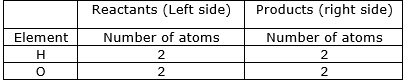### Question 9. Explain the following terms with examples. Balanced equation

Question 9.

Explain the following terms with examples.

Balanced equation